# Complexity-theoretic aspects of expanding cellular automata

## Abstract

The expanding cellular automata (XCA) variant of cellular automata is investigated and characterized from a complexity-theoretical standpoint. An XCA is a one-dimensional cellular automaton which can dynamically create new cells between existing ones. The respective polynomial-time complexity class is shown to coincide with $${\le _{tt}^p}(\textsf {NP})$$, that is, the class of decision problems polynomial-time truth-table reducible to problems in $$\textsf {NP}$$. An alternative characterization based on a variant of non-deterministic Turing machines is also given. In addition, corollaries on select XCA variants are proven: XCAs with multiple accept and reject states are shown to be polynomial-time equivalent to the original XCA model. Finally, XCAs with alternative acceptance conditions are considered and classified in terms of $${\le _{tt}^p}(\textsf {NP})$$ and the Turing machine polynomial-time class $$\textsf {P}$$.

## Introduction

Traditionally, cellular automata (CAs) are defined as a rigid and immutable lattice of cells; their behavior is dictated exclusively by a local transition function operating on homogeneous local configurations. This can be generalized, for instance, by mutable neighborhoods (Rosenfeld and Wu 1981) or by endowing CAs with the ability to shrink, that is, delete their cells (Rosenfeld et al. 1983). When shrinking, the automaton’s structure and dimension are preserved by “gluing” the severed parts and reconnecting their delimiting cells as neighbors. When employed as language recognizers, shrinking CAs (SCAs) can be more efficient than standard CAs (Rosenfeld et al. 1983; Kutrib et al. 2015).

Other variants of CAs with dynamically reconfigurable neighborhoods have emerged throughout the years. In the case of two-dimensional CAs, there is the structurally dynamical CA (SDCA) due to Ilachinski and Halpern (1987), in which the connections between neighbors are created and dropped depending on the local configuration. In the one-dimensional case, further variants in this sense are considered in the work of Dubacq (1994), where one finds, in particular, CAs whose neighborhoods vary over time. Dubacq also proposes the dynamically reconfigurable CA (DRCA), a CA whose cells are able to exchange their neighbors for neighbors of their neighbors. Dantchev (2008) later points out a drawback in the definition of DRCAs and proposes an alternative dubbed the dynamic neighborhood CA (DNCA).

By relaxing the arrangement of cells as a lattice, CAs may be generalized to graphs (Tomita et al. 2002). Graph automata are related to CAs in that each vertex in the graph corresponds to a cell; thus, graphs whose vertices have finite degrees provide a natural generalization of CAs. Tomita et al. (2002) also define a rule based on topological refinements of graphs, which may be used as a model for biological cell division. An additional example of cell division in this sense is the “inflating grid” of Arrighi and Dowek (2013).

Modeling cell division and growth, in fact, was one of the driving motivations towards the investigation of the expanding CA (XCA) in Modanese (2016). An XCA is, in a way, the opposite of an SCA; instead of cells vanishing, new cells can emerge between existing ones. This operation is topologically similar to the cell division of graph automata; as in the SCA model, however, it maintains the overall arrangement and connectivity of the automaton’s cells as similar as possible to that of standard CAs (i.e., a bi-infinite, one-dimensional array of cells).

We mention a few aspects in which XCAs differ from the aforementioned variants. Contrary to SDCAs or CAs with dynamic neighborhoods such as DRCAs and DNCAs, XCAs enable the creation of new cells, not simply new links between existing ones. In addition, the XCA model does not focus as much on the reconfiguration of cells; in it, the neighborhoods are homogeneous and predominantly immutable. Furthermore, in contrast to the far more general graph automata, XCAs are still one-dimensional CAs; this ensures basic CA techniques (e.g., synchronization) function the same as they do in standard CAs.

Finally, shrinking and expanding are not mutually exclusive. Combining them yields the shrinking and expanding CA (SXCA). The polynomial-time class of SXCA language deciders was shown to coincide with $$\textsf {PSPACE}$$ (Modanese 2016; Modanese and Worsch 2016).

A previous result by Modanese (2016) is that, for the class $$\textsf {XCAP}$$ of polynomial-time XCA language deciders, we have $$\textsf {NP}\cup \textsf {coNP}\subseteq \textsf {XCAP}\subseteq \textsf {PSPACE}$$. A precise characterization of $$\textsf {XCAP}$$, however, remained outstanding. Such was the topic of the author’s master’s thesis (Modanese 2018), the results of which are summarized in this paper. The main result (Theorem 8) is $$\textsf {XCAP}$$ being equal to the class of decision problems which are polynomial-time truth-table reducible to $$\textsf {NP}$$, denoted $${\le _{tt}^p}(\textsf {NP})$$.

The rest of the paper is organized as follows: Sect. 2 covers the fundamental definitions and results needed for the subsequent discussions. Following that, Sect. 3 recalls the main result of Modanese (2016) concerning $$\textsf {XCAP}$$ and presents two characterizations of $$\textsf {XCAP}$$, one based on $${\le _{tt}^p}(\textsf {NP})$$ (Theorem 8) and another (Theorem 14) based on a variant of non-deterministic Turing machines (NTMs). Section 4 covers some immediate corollaries, in particular by considering an XCA variant with multiple accept and reject states as well as two other variants with diverse acceptance conditions. Finally, Sect. 5 concludes.

This is an extended and revised version of a preliminary paper presented at the AUTOMATA 2019 (Modanese 2019). Section 3 has been expanded to provide a complete proof of Proposition 7 (instead of only an outline), which is now found in Sect. 3.1, while the material in Sect. 3.3 is entirely novel. Other improvements include a full proof of Lemma 5, an updated abstract, broader discussions of concepts and results, and minor text edits.

## Definitions

This section recalls basic concepts and results needed for the proofs and discussions in the later sections and is broken down in two parts. The first is concerned with basic topics regarding formal languages, Turing machines, and Boolean formulas. The second part covers the definition of expanding CAs.

### Formal languages and turing machines

It is assumed the reader is familiar with the fundamentals of cellular automata and complexity theory (see, e.g., standard references such as Delorme and Mazoyer 1999 and Arora and Barak 2009). Unless stated otherwise, all words have length at least one. For sets A and B, $$B^A$$ denotes the set of functions $$A \rightarrow B$$. For an alphabet $$\varSigma$$, $$\varSigma ^*$$ is the set of words over $$\varSigma$$. A $$\omega \omega$$-word is a biinfinite word $$w\in \varSigma ^{\mathbb {Z}}$$. The notion of a complete language is employed strictly in the sense of polynomial-time many-one reductions by deterministic Turing machines.

#### Boolean formulas

Let V be a language of variables over an alphabet $$\varSigma$$ which, without loss of generality, is disjoint from $$\{F, T, \lnot , \wedge , \vee , (, )\}$$. $$\textsf {BOOL}_V$$ denotes the formal language of Boolean formulas over the variables of V. For better readability, we shall prefer prefix notation when writing out formulas (e.g., $$\wedge ( f, g )$$ for formulas f and g instead of the more common infix notation $$f \wedge g$$).

An interpretation of V is a map $$I:V \rightarrow \{F, T\}$$. Each such I gives rise to an evaluation $$E_I:\textsf {BOOL}_V \rightarrow \{F, T\}$$ which, given a formula $$f \in \textsf {BOOL}_V$$, substitutes each variable $$x \in V$$ with the truth value I(x) and reduces the resulting formula using standard propositional logic. A formula f is satisfiable if there is an interpretation I such that $$E_I(f) = T$$, and f is a tautology if this holds for every I.

In order to define the languages $$\textsf {SAT}$$ of satisfiable formulas and $$\textsf {TAUT}$$ of tautologies, a language V of variables must first be agreed on. In this paper, variables are encoded as binary strings prefixed by a special symbol x, that is, $$V = \{x\} \cdot \{0, 1\}^+$$. The language $$\textsf {SAT}$$ contains exactly the satisfiable formulas of $$\textsf {BOOL}_V$$. Similarly, $$\textsf {TAUT}$$ contains exactly the tautologies of $$\textsf {BOOL}_V$$. The following is a classical result concerning $$\textsf {SAT}$$ and $$\textsf {TAUT}$$:

### Theorem 1

(Cook 1971) $$\textsf {SAT}$$ is $$\textsf {NP}$$-complete, and $$\textsf {TAUT}$$ is $$\textsf {coNP}$$-complete.

#### Truth-table reductions

The theory of truth-table reductions was established by Ladner et al. (1975) and Ladner and Lynch (1976). Later, Wagner (1990) showed the class of decision problems polynomial-time truth-table (i.e., Boolean-circuit) reducible to $$\textsf {NP}$$, denoted $${\le _{tt}^p}(\textsf {NP})$$, remains the same even if the reduction is in terms of Boolean formulas (instead of circuits). We refer to Buss and Hay (1991) for a series of alternative characterizations of $${\le _{tt}^p}(\textsf {NP})$$. The inclusions $$\textsf {NP}\cup \textsf {coNP}\subseteq {\le _{tt}^p}(\textsf {NP})$$ and $${\le _{tt}^p}(\textsf {NP})\subseteq \textsf {PSPACE}$$ are known to hold.

A more formal treatment of the class $${\le _{tt}^p}(\textsf {NP})$$ is not necessary to establish the results of this paper; it suffices to note $${\le _{tt}^p}(\textsf {NP})$$ has complete languages. In particular, we are interested in Boolean formulas with $$\textsf {NP}$$ and $$\textsf {coNP}$$ predicates. To this end, we employ $$\textsf {SAT}$$ and $$\textsf {TAUT}$$ to define membership predicates of the form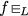, where f is a Boolean formula, $$L \in \{ \textsf {SAT}, \textsf {TAUT}\}$$, and “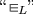” is a purely syntactic construct which stands for the statement “$$f \in L$$”.

### Definition 2

($$\textsf {SAT}^\wedge \text {-}\textsf {TAUT}^\vee$$) Let $$V = \{x\} \cdot \{0,1\}^+$$ and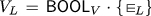for $$L \in \{\textsf {SAT}, \textsf {TAUT}\}$$. The language $$\textsf {BOOL}^{\wedge \vee }_{\textsf {SAT},\textsf {TAUT}} \subseteq \textsf {BOOL}_{V_\textsf {SAT}\cup V_\textsf {TAUT}}$$ is defined recursively as follows:

1. 1.

$$V_\textsf {SAT}, V_\textsf {TAUT}\subseteq \textsf {BOOL}^{\wedge \vee }_{\textsf {SAT},\textsf {TAUT}}$$.

2. 2.

For $$v \in V_\textsf {SAT}$$ and $$f \in \textsf {BOOL}^{\wedge \vee }_{\textsf {SAT},\textsf {TAUT}}$$, $$\wedge (v, f) \in \textsf {BOOL}^{\wedge \vee }_{\textsf {SAT},\textsf {TAUT}}$$.

3. 3.

For $$v \in V_\textsf {TAUT}$$ and $$f \in \textsf {BOOL}^{\wedge \vee }_{\textsf {SAT},\textsf {TAUT}}$$, $$\vee (v, f) \in \textsf {BOOL}^{\wedge \vee }_{\textsf {SAT},\textsf {TAUT}}$$.

The language $$\textsf {SAT}^\wedge \text {-}\textsf {TAUT}^\vee \subseteq \textsf {BOOL}^{\wedge \vee }_{\textsf {SAT},\textsf {TAUT}}$$ contains all formulas which are true under the interpretation mappingto the truth value of the statement “$$f \in L$$”.

For example, given $$f_1, f_2, f_3, f_4 \in \textsf {BOOL}_V$$, the following formula f is in $$\textsf {BOOL}^{\wedge \vee }_{\textsf {SAT},\textsf {TAUT}}$$: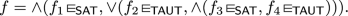Then, $$f \in \textsf {SAT}^\wedge \text {-}\textsf {TAUT}^\vee$$ if, for instance, $$f_1 \in \textsf {SAT}$$ and $$f_2 \in \textsf {TAUT}$$ holds.

From the results of Buss and Hay (1991) it follows:

### Theorem 3

$$\textsf {SAT}^\wedge \text {-}\textsf {TAUT}^\vee$$ is $${\le _{tt}^p}(\textsf {NP})$$ -complete.

### Cellular automata

Here, we are strictly interested in one-dimensional cellular automata (CAs) with the standard neighborhood and employed as language deciders. CA deciders possess a quiescent state q; cells which are not in this state are said to be active and may not become quiescent. The input for a CA decider is provided in its initial configuration surrounded by quiescent cells. As deciders, CAs are Turing complete, and, more importantly, CAs can simulate TMs in real-time (Smith 1971). Conversely, it is known a TM can simulate a CA with time complexity t in time at most $$t^2$$. A corollary is that the class of problems decidable in polynomial time by CAs is exactly $$\textsf {P}$$.

#### Expanding cellular automata

First considered in Modanese (2016), the expanding CA (XCA) is similar to the shrinking CA (SCA) in that it is dynamically reconfigurable; instead of cells being deleted, however, in an XCA new cells emerge between existing ones. This does not alter the underlying topology, which remains one-dimensional and biinfinite.

For modeling purposes, the new cells are seen as hidden between the original (i.e., visible) ones, with one hidden cell placed between any two neighboring visible cells. These latter cells serve as the hidden cell’s left and right neighbors and are referred to as its parents. In each CA step, a hidden cell observes the states of its parents and either assumes a non-hidden state, thus becoming visible, or remains hidden. In the former case, the cell assumes the position between its parents and becomes an ordinary cell (i.e., visible), and the parents are reconnected so as to adopt the new cell as a neighbor. Visible cells may not become hidden, and we refer to hidden cells neither as active nor as quiescent (i.e., we treat them as a tertium quid).

### Definition 4

(XCA) Let $$N = \{ -1, 0, 1 \}$$ be the standard neighborhood. An expanding CA (XCA) is a CA A with state set Q and local transition function $$\delta :Q^N \rightarrow Q$$ and which possesses a distinguished hidden state $$\odot \in Q$$. For any local configuration $$\ell :N \rightarrow Q$$, $$\delta (\ell ) = \odot$$ is allowed only if $$\ell (0) = \odot$$.

For a global configuration $$c:{\mathbb {Z}}\rightarrow Q$$, let $$h_c:{\mathbb {Z}}\rightarrow Q^N$$ be such that $$h_c(z)(-1) = c(z)$$, $$h_c(z)(0) = \odot$$, and $$h_c(z)(1) = c(z + 1)$$ for any $$z \in {\mathbb {Z}}$$. Define $$\alpha :Q^{\mathbb {Z}}\rightarrow Q^{\mathbb {Z}}$$ as follows, where $$\varDelta$$ is the standard CA global transition function (as induced by $$\delta$$):

\begin{aligned} \alpha (c)(z) = {\left\{ \begin{array}{ll} \varDelta (c)(\frac{z}{2}), &{} \text {{ z} even} \\ \delta (h_c(\frac{z-1}{2})), &{} \text {otherwise.} \end{array}\right. } \end{aligned}

Finally, with c still arbitrary, let $$\varPhi :Q^{\mathbb {Z}}\rightarrow Q^{\mathbb {Z}}$$ be the mapFootnote 1 that acts as a homomorphism on c deleting any occurrence of $$\odot$$ (and contracting the remaining states towards zero), formally:

\begin{aligned} \varPhi (c)(z) = {\left\{ \begin{array}{ll} c(m_+(z)), &{} z \ge 0 \\ c(m_-(-z-1)), &{} \text {otherwise} \end{array}\right. } \end{aligned}

where $$m_+(z)$$ is the maximum $$i \in {\mathbb {Z}}$$ for which $$| \{ j \in [0,i) \mid c(j) \ne \odot \} | = z$$ and $$m_-(z)$$ is the minimum $$i \in {\mathbb {Z}}$$ for which $$| \{ j \in (i,-1] \mid c(j) \ne \odot \} | = z$$. Then the global transition function of A is $$\varDelta ^\text {X}= \varPhi \circ \alpha$$.

Figure 1 illustrates an XCA A and its operation for input 001010 as an example. The local transition function $$\delta$$ of A is as follows:

\begin{aligned} \delta (q_{-1}, q_0, q_1) = {\left\{ \begin{array}{ll} q_{-1} \oplus q_1, &{} q_{-1}, q_1 \in \{0,1\} \\ q_0, &{} \text {otherwise} \end{array}\right. } \end{aligned}

where $$\oplus$$ denotes the bitwise XOR operation, that is, addition modulo 2. The initial configuration is marked as c. The hidden cells are those in state $$\odot$$. Starting from c, $$\alpha$$ applies $$\delta$$ to each local configuration, where $$h_c$$ specifies the local configurations for the hidden cells; $$\alpha$$ also promotes all originally hidden cells to visible ones. Finally, $$\varPhi$$ then eliminates cells having the state $$\odot$$, as such cells are per definition not allowed to be visible (rather, they are present only implicitly in the global configuration).

The supply of hidden cells is never depleted; whenever a hidden cell becomes visible, new hidden cells appear between it and its neighbors. Thus, the number of active cells in an XCA may increase exponentially:

### Lemma 5

Let A be an XCA. For an input of size nA has at most $$a(t) = (n + 3)2^t - 3$$ active cells after $$t \in {\mathbb {N}}_0$$ steps. This upper bound is sharp.

### Proof

The claim is proven using induction on $$t \in {\mathbb {N}}_0$$. The induction basis is evident since A has exactly n active cells in time step $$t = 0$$. For the induction step, assume the claim holds for some $$t \in {\mathbb {N}}_0$$. Without loss of generality, it may also be assumed that the number of active cells in A is maximal (i.e., equal to a(t)). Then A can have at most $$2a(t) + 3$$ active cells in time step $$t + 1$$ since a(t) many cells were already active, and a maximum of two quiescent and $$a(t) + 1$$ hidden cells may become active in the transition to the next step. The proof is complete by using $$2a(t) + 3 = (n+3)2^{t+1} -3 = a(t+1)$$. $$\square$$

We have postponed defining the acceptance condition for XCAs until now. Usually, a CA possesses a distinguished cell, often cell 0, which dictates the automaton’s accept or reject response (Kutrib 2009). In the case of XCAs, however, under a reasonable complexity-theoretical assumption (i.e., $$\textsf {P}\ne {\le _{tt}^p}(\textsf {NP})$$) such an acceptance condition results in XCAs not making full use of the efficient cell growth indicated in Lemma 5 (see Sect. 4.3). This phenomenon does not occur if the acceptance condition is defined based on unanimity, that is, in order for an XCA to accept (or reject), all its cells must accept (or reject) simultaneously. This acceptance condition is by no means novel (Rosenfeld 1979; Sommerhalder and van Westrhenen 1983; Ibarra et al. 1985; Kim and McCloskey 1990). As an aside, note all (reasonable) CA time complexity classes (including, in particular, linear- and polynomial-time) remain invariant when using this acceptance condition instead of the standard one.

Also of note is that, for the standard acceptance condition, we insist on unique accept and reject states. This serves to not only simplify some arguments in Sect. 3 but also to show that unique states already suffice to decide problems in $${\le _{tt}^p}(\textsf {NP})$$. We revisit this topic in Sect. 4.1, where we consider XCAs with multiple accept and reject states (and prove that the class of problems that can be decided efficiently remains the same).

### Definition 6

(Acceptance condition, time complexity) Each XCA has a unique accept state a and a unique reject state r. An XCA A halts if all active (and visible) cells are either all in state a, in which case the XCA accepts, or they are all in state r, in which case it rejects; if neither is the case, the computation continues. L(A) denotes the set of words accepted by A.

The time complexity of an XCA (for an input w) is the number of elapsed steps until it halts. An XCA decider is an XCA which halts on every input. A language L is in $$\textsf {XCAP}$$ if there is an XCA decider $$A'$$ with polynomial time complexity (in the length |w| of w) and such that $$L = L(A')$$.

In summary, the decision result of an XCA decider is the one indicated by the first configuration in which its active cells are either all in the accept or all in the reject state. This agrees with our aforementioned notion of a unanimous decision.

## Characterizing $$\textsf {XCAP}$$

This section covers the main result of this paper, that is, characterizing $$\textsf {XCAP}$$ as being equal to $${\le _{tt}^p}(\textsf {NP})$$ (Theorem 8). It is subdivided into three parts: First, we address a result from Modanese (2016) which is relevant towards proving the aforementioned characterization. Next, we state and prove Theorem 8. Finally, we discuss an alternative characterization of $$\textsf {XCAP}$$ based on NTMs.

### An XCA for $$\textsf {TAUT}$$

In this section, we cover the following result from Modanese (2016), which provides the starting point towards proving Theorem 8:

### Proposition 7

$$\textsf {NP}\cup \textsf {coNP}\subseteq \textsf {XCAP}$$.

Since many-one reductions by TMs can be simulated by (X)CAs in real-time, it suffices to show $$\textsf {XCAP}$$ contains $$\textsf {NP}$$- and $$\textsf {coNP}$$-complete problems. We construct XCAs for $$\textsf {SAT}$$ and $$\textsf {TAUT}$$ which run in polynomial time and apply Theorem 1. Since acceptance and rejection are defined symmetrically (as per Definition 6), if L can be accepted by an XCA A, then swapping the accept and reject states of A we obtain an XCA that decides the complement of L with the exact same complexity as A. Hence, as $$\textsf {NP}$$ and $$\textsf {coNP}$$ are complementary classes, it suffices to show $$\textsf {coNP}\subseteq \textsf {XCAP}$$.

The key idea towards the result is that an XCA can efficiently duplicate portions of its configuration: Let a block denote a subconfiguration $$\# w \#$$ where $$\#$$ is a (special) separator symbol and w is a word not containing $$\#$$. In particular, starting from any such block, the XCA can, in a single step, produce the block $$\# w_2 \ \#$$ where is a separator symbol different from $$\#$$ and \begin{aligned} w_2 = (w(0),0) (w(0),1) \cdots (w(|w| - 1),0) (w(|w| - 1),1) \end{aligned} duplicates the word w (i.e., we have $$|w_2| = 2|w|$$). Using a stable sorting algorithm (following, e.g., techniques from Gordillo and Luna (1994)), the XCA then sorts the symbols into place according to the second component of the tuples above and obtains the subconfiguration $$\# w \ w \#$$ in a linear number of steps. ### Proof In accordance with the previous discussion, we construct an XCA A for $$\textsf {TAUT}$$. Firstly, A verifies the input f is a syntactically correct formula; this can be done, for instance, simply by simulating a TM for this task. Following that, the operation of A can be subdivided into two large steps. In the first of them, A iteratively expands its configuration (in a way we shall describe in more detail) so as to cover every possible truth assignment of the variables of f and arrives at a configuration $$c_f$$ (detailed below). The second step starts from $$c_f$$, computes the evaluations of f under the respective truth assignments in parallel, and accepts or rejects according to whether the results are all “true” or not. Both procedures require time polynomial in the length |f| of f; thus, A runs in polynomial time. Step 1 Given a Boolean formula f over m variables, let $$x_0, \dots , x_{m-1}$$ be the ordering of its variables according to their first appearance in f when this is read from left to right. Furthermore, letting $$s_0, \dots , s_{2^m - 1}$$ be the lexicographic ordering of the strings in $$\{ F, T \}^m$$ (under the convention that F precedes T), we obtain a natural ordering $$I_0, \dots , I_{2^m - 1}$$ of the $$2^m$$ possible interpretations of the $$x_i$$ by identifying each $$s_j$$ with the interpretation $$I_j$$ that satisfies $$I_j(x_i) = s_j(i)$$. We let $$c_f$$ be the following configuration: \begin{aligned} \cdots \; q \; \# \; (E_0)^{|f|} \; \# \; \cdots \; \# \; (E_{2^m - 1})^{|f|} \; \# \; q \; \cdots \end{aligned} where $$E_j = E_{I_j}(f)$$ is the evaluation of f under $$I_j$$ (as a symbol, i.e., an element of the alphabet $$\{ F, T \}$$). We now further specify how $$c_f$$ is reached. A starts by surrounding f with $$\#$$ delimiters. Each block $$\# f \#$$ of A repeats the following procedure as long as f contains at least one variable: 1. 1. Duplicate f (as described previously), yielding the subconfiguration $$\# f \ f' \#$$. 2. 2. Determine the first variable $$v = xy$$ in f, where $$y \in \{ 0,1 \}^+$$, and replace every occurrence of v in f (resp., $$f'$$) with $$F^k$$ (resp., $$T^k$$), where $$k = |v| = 1 + |y|$$. 3. 3. Replace the middle delimiter with $$\#$$ and synchronize the two blocks corresponding to f and $$f'$$ (so they continue their operation at the same time).

When f no longer contains a variable, the block evaluates it directly (e.g., by simulating a TM for this task).

The correctness of the above is shown by induction on m. The case $$m = 0$$ is trivial, so assume $$m > 0$$. The above procedure replaces the variables of f such that precisely $$2^m$$ copies are produced, each corresponding to an $$I_j$$ (and according to the ordering described above). Also note the blocks of A always have the same length and, because of step 3 above and using transitivity, any two blocks are synchronized with each other. Thus, when f has no variables left, the evaluations all happen and terminate at the same time, thus producing the desired configuration. Finally, it is straightforward to show the above procedure requires polynomial time.

Step 2 We now describe the second procedure of A which, starting from the configuration $$c_f$$ of, leads A to accept or reject depending on the results present in $$c_f$$.

Notice that, in the first step above, we ensured that $$c_f$$ is reached in such a way that the blocks corresponding to the $$E_i$$ are all synchronized. Hence, from this point each block (including the delimiting $$\#$$ cells) initiates a synchronization, following which all cells in the block simultaneously enter the accept (resp., reject) state if the respective evaluation’s result was T (resp., F). The reject state is maintained while accept states yield to a reject state, that is, we have $$\delta (q_1, r, q_2) = r$$ and $$\delta (q_1, a, q_2) = r$$ for the local transition function $$\delta$$ of A and arbitrary states $$q_1$$ and $$q_2$$. Thus, if all evaluations are “true” (i.e., their result is T), the cells all simultaneously enter the accept state; otherwise, all cells necessarily enter the reject state. Since this process also takes only polynomial time, the claim follows. $$\square$$

We conclude this section by stressing that step 2 above builds on a “trick” that is only possible due to the unanimous acceptance condition of XCAs. Assume, for the moment, that the XCA A of before can continue computing (i.e., does not halt) even if it has reached a configuration in which it accepts or rejects. Then A is guaranteed to eventually reach a configuration in which it rejects regardless of what the results for the evaluations of f are. This means the only case in which A is prevented from rejecting is when it accepts (namely when all of the $$E_i$$ are “true”); that is, A is capable of rejecting under the condition it has not accepted. This kind of behavior is quite different from, say, an NTM (seen as an alternating Turing machine with only existential states), where the result of each computation branch is completely independent of the other branches. We shall come back to this point later in Sect. 3.3 and address it from another perspective.

### A first characterization

In this section, we prove the main result of this paper:

### Theorem 8

$$\textsf {XCAP}= {\le _{tt}^p}(\textsf {NP})$$.

The equality in Theorem 8 is proven by considering the two inclusions (Propositions 9 and 12).

### Proposition 9

$${\le _{tt}^p}(\textsf {NP})\subseteq \textsf {XCAP}$$.

### Proof

The claim is shown by constructing an XCA A that decides $$\textsf {SAT}^\wedge \text {-}\textsf {TAUT}^\vee$$ (see Definition 2 and Theorem 3) in polynomial time. The actual inclusion follows from the fact that CAs can simulate polynomial-time many-one reductions by TMs in real-time.

Given a problem instance f, A evaluates f recursively. Without loss of generality, we may assume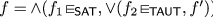, where $$f'$$ is a further problem instance; other instances of $$\textsf {SAT}^\wedge \text {-}\textsf {TAUT}^\vee$$ are obtained by replacing $$f_1$$, $$f_2$$, or $$f'$$ with a trivial formula (e.g., a trivial tautology).

To evaluate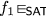, A emulates the behavior of the XCA for $$\textsf {SAT}$$ (see Proposition 7); however, special care must be taken to ensure A does not halt prematurely. All computation branches retain a copy of f. Whenever a branch obtains a “true” result, the respective cells do not directly accept (as in the original construction); instead, they proceed with evaluating the formula’s next connective. Conversely, if the result is false, the respective cells simply enter the reject state. The behavior for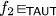is analogous, with A emulating the XCA for $$\textsf {TAUT}$$ instead (and with exchanged accept and reject states, accordingly). Additionally, we require $$\delta (q_1, r, q_2) = a$$ and $$\delta (q_1, a, q_2) = r$$ for every states $$q_1$$ and $$q_2$$, that is, once a cell enters the accept or reject state, it is forced to unconditionally alternate between the two. To ensure A is still able to accept or reject, we (arbitrarily) enforce accept states only exist in even-numbered steps and reject states only in odd-numbered ones.Footnote 2

If $$f_1 \not \in \textsf {SAT}$$, all branches of A transition into the reject state, and A rejects. Otherwise, $$f_1$$ is satisfiable; thus, at least one branch obtains a “true” result, and A continues to evaluate f until the (aforementioned) base case is reached. An analogous argument applies for $$f_2$$. Note the synchronicity of the branches guarantee they operate exactly the same and terminate at the same time. The repeated transition between accept and reject states guarantee the only cells relevant for the final decision of A are those in the branches which are still “active” (in the sense they are still evaluating f).

In conclusion, A accepts f if and only if it evaluates to true; otherwise, A rejects f. A runs in polynomial time since f has at most |f| predicates and since evaluating a predicate requires polynomial time in |f|. $$\square$$

For the converse, we express an XCA computation as a $$\textsf {SAT}^\wedge \text {-}\textsf {TAUT}^\vee$$ instance. The main effort here lies in defining the appropriate “variables”:

### Definition 10

($$\textsf {STATE}_\forall$$) Let A be an XCA, and let $$V_A$$ be the set of triples (wtz), w being an input for A, $$t \in \{0, 1\}^+$$ a (standard) binary representation of $$\tau \in {\mathbb {N}}_0$$, and z a state of A. $$\textsf {STATE}_\forall (A) \subseteq V_A$$ is the subset of triples such that, if A is given w as input, then after $$\tau$$ steps all active cells are in state z.

### Lemma 11

For any XCA A with polynomial time complexity, $$\textsf {STATE}_\forall (A) \in \textsf {coNP}.$$

### Proof

Let $$p:{\mathbb {N}}_+\rightarrow {\mathbb {N}}_0$$ be a polynomial bounding the time complexity of A, that is, for an input of size n, A always terminates after at most p(n) many steps. Suppose there is an NTM T which covers all active cells in step $$\tau$$ of A for input w, that is, for each such active cell r there is at least one computation branch of T corresponding to r. Furthermore, assume T can then compute the state $$z'$$ of r in polynomial time and accepts if and only if $$z' = z$$. Without restriction, we may assume $$\tau \le p(|w|)$$; this can be enforced by T, for instance, by computing p(|w|) and rejecting whenever $$\tau > p(|w|)$$. Then, the claim follows immediately from the existence of T: If all computation branches of T accept, then in step $$\tau$$ all cells of A are in state z; otherwise, there is a cell in a state which is not z, and T rejects.

The rest of the proof is concerned with the construction of a T with the properties just described. First, we describe the construction, followed by arguing it has the desired complexity (which is fairly straightforward). The last part of the proof concerns the correctness of T which, although fairly evident, calls for a more technical argument.Footnote 3

Construction To compute the state of a (in particular, active) cell in step $$\tau$$, T computes a series of subconfigurations $$c_0, \dots , c_\tau$$ of A, that is, contiguous excerpts of the global configuration of A. As the number of cells in an XCA may increase exponentially in the number of computation steps, bounding $$c_i$$ is essential to ensure T runs in polynomial time; in particular, T maintains $$|c_i| = 1 + 2(\tau - i)$$ (for $$i \ge 1$$), thus ensuring the lengths of the $$c_i$$ are linear in $$\tau$$ (which, in turn, is polynomial in |w|). This choice of length for the $$c_i$$ ensures each of the subconfigurations correspond to a cell of A surrounded by $$\tau - i$$ cells on either side (i.e., each $$c_i$$ corresponds to the extended neighborhood of radius $$\tau - i$$ of said cell). The non-determinism of T is used exclusively in picking the cells from $$c_i$$ which are to be included in the next subconfiguration $$c_{i+1}$$.

The initial subconfiguration $$c_0$$ is set to be $$q^{2\tau } w q^{2\tau }$$, thus containing the input word as well as (as shall be proven) a sufficiently large number of surrounding quiescent cells. To obtain $$c_{i+1}$$ from $$c_i$$, T applies the transition function of A to $$c_i$$ and obtains a new temporary subconfiguration $$c_{i+1}'$$. The next state of the two “boundary” cells (i.e, those belonging to indices 0 and $$|c_i| - 1$$) cannot be determined, and they are excluded from $$c_{i+1}'$$. As a result, $$c_{i+1}'$$ contains $$|c_i| - 2$$ cells from the previous configuration $$c_i$$, plus a maximum of $$|c_i| - 1$$ additional cells which were previously hidden. Therefore, to maintain $$|c_{i+1}| = 1 + 2(\tau - (i+1))$$, T non-deterministically sets $$c_{i+1}$$ to a contiguous subset of $$c_{i+1}'$$ containing exactly $$1 + 2(\tau - (i+1)) \le |c_i| - 2$$ cells.

The process of selecting a next subconfiguration $$c_{i+1}$$ from $$c_i$$ is depicted in Fig. 2. In the illustration, $$|c_i|$$ has been replaced with n for legibility. T at first applies the global transition function of A to obtain an intermediate subconfiguration $$c_{i+1}'$$ with $$m = |c_{i+1}'|$$ cells. Because of hidden cells, $$c_{i+1}'$$ may consist of $$n - 2 \le m \le 2n - 3$$ cells. Non-determinism is used to select a contiguous subconfiguration of $$n - 2$$ cells, thus giving rise to $$c_{i+1}$$.

Complexity T runs in polynomial time since the invariant $$|c_i| = 1 + 2(\tau - i)$$ guarantees the number of states T computes in each step is bounded by a multiple of $$\tau$$, which, in turn, we assumed to be bounded by p(|w|). Only |w| has to be taken into account when estimating the time complexity of T since the encoding of z is O(1) long, while that of t has length $$O(\log p(|w|)) = O(\log |w|)$$; as a result, the problem instance (wtz) has length O(|w|).

Correctness To show T covers all active cells of A in step $$\tau$$, it suffices to prove the following by induction: Let $$i \in \{ 0, \dots , \tau \}$$, and let $$z_1, \dots , z_m$$ be the active cells of A in step i; then T covers all subconfigurations of $$q^{2(\tau - i)} z_1 \cdots z_m q^{2(\tau - i)}$$ of size $$1 + 2(\tau - i)$$, that is, for every such subconfiguration s there is a branch of T in which it picks s as its $$c_i$$. Note this corresponds to T covering all subconfigurations of A in step i which contain at least one active cell; thus, when T reaches step $$\tau$$, it covers all subconfigurations of $$z_1 \cdots z_m$$ of size 1, that is, all active cells.

The induction basis follows from $$c_0 = q^{2\tau } w q^{2\tau }$$. For the induction step, fix a step $$0 < i \le \tau$$ and assume the claim holds for all steps prior to i. To each subconfiguration of $$q^{2(\tau - i)} z_1 \cdots z_m q^{2(\tau - i)}$$ having size $$1 + 2(\tau - i)$$ corresponds a cell r which is located in its center; thus, we may unambiguously denote every such subconfiguration by $$s_i(r)$$. Now let $$s_i(r)$$ be given and consider the following three cases: r was active in step $$i - 1$$; r was a hidden cell which became active in the transition to step i; or r was a quiescent cell in step $$i - 1$$ and, since $$|s_i(r)| = 1 + 2(\tau - i)$$ and r is the middle cell of $$s_i(r)$$, r is at most $$\tau - i$$ cells away from $$z_1$$ or $$z_m$$.

In the first case, by the induction hypothesis, there is a value of $$c_{i-1}$$ corresponding to $$s_{i-1}(r)$$; since only the two boundary cells are present in $$c_{i-1}$$ but not in $$c_i'$$, T can choose $$c_i$$ from $$c_i'$$ with r as its middle cell and obtain $$s_i(r)$$. In the second case, for any of the two parents $$p_1$$ and $$p_2$$ of r, there are, by the induction hypothesis, values of $$c_{i-1}$$ which equal $$s_{i-1}(p_1)$$ and $$s_{i-1}(p_2)$$; in either case, choosing $$c_i$$ from $$c_i'$$ with r as its middle cell again yields $$s_i(r)$$.

Finally, if r was a quiescent cell, then, without loss of generality, consider the case in which r was located to the left of the active cells in step $$i - 1$$. By the induction hypothesis, for each cell $$r'$$ up to $$\tau - i + 1$$ cells away from the leftmost active cell $$z_1$$ there is a value of $$c_{i-1}$$ corresponding to $$s_{i-1}(r')$$, and the first case applies; the only exception is if $$c_i$$ would then contain only quiescent cells, in which case r would be located strictly more than $$\tau - i$$ cells away from $$z_1$$, thus contradicting our previous assumption. The claim follows. $$\square$$

### Proposition 12

$$\textsf {XCAP}\subseteq {\le _{tt}^p}(\textsf {NP})$$.

### Proof

Let $$L \in \textsf {XCAP}$$, and let A be an XCA for L whose time complexity is bounded by a polynomial $$p:{\mathbb {N}}_+\rightarrow {\mathbb {N}}_0$$. Additionally, let w be an input for A, $$V_A$$ be as in Definition 10, and let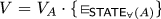, where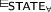is a syntactic symbol standing for membership in $$\textsf {STATE}_\forall (A)$$ (cf. Definition 2). Define $$f_0(w), \dots , f_{p(n)}(w) \in \textsf {BOOL}_V$$ recursively by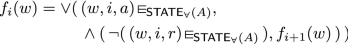for $$i < p(n)$$ and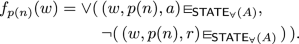Lemma 11 together with the $$\textsf {coNP}$$-completeness of $$\textsf {TAUT}$$ (see Theorem 1) ensures each subformula of the form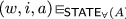is polynomial-time many-one reducible to an equivalent (in the sense of evaluating to the same truth value under the respective interpretations; see Definition 2) $$\textsf {SAT}^\wedge \text {-}\textsf {TAUT}^\vee$$ formula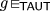, g being a $$\textsf {TAUT}$$ instance. Similarly, each subformula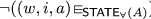is reducible to an equivalent formula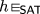. Since each of the $$f_i(w)$$ may contain only polynomially (respective to |w|) many connectives, each is polynomial-time (many-one) reducible to an equivalent $$\textsf {SAT}^\wedge \text {-}\textsf {TAUT}^\vee$$ instance $$f_i'(w)$$.

By the definition of XCA (i.e., Definitions 4 and 6) and our choice of p, $$f'(w) = f_0'(w)$$ is true if and only if A accepts w. Since $$f'(w)$$ is such that $$|f'(w)|$$ is polynomial in |w|, this provides a polynomial-time (many-one) reduction of L to a problem instance of $$\textsf {SAT}^\wedge \text {-}\textsf {TAUT}^\vee \in {\le _{tt}^p}(\textsf {NP})$$. The claim follows. $$\square$$

This concludes the proof of Theorem 8.

### A turing machine characterization

We now turn to a closer investigation of the relation between XCA polynomial-time computations and the class $${\le _{tt}^p}(\textsf {NP})$$. In this section, we shall view NTMs as a special case of alternating Turing machines (ATMs), that is, as possessing a computation tree in which all branches are existential. Recall the computational strategy of the XCA in Proposition 7 essentially consists of creating multiple computation branches, each corresponding to a possible variable assignment of the input formula. In a sense, this merely replicates the standard NTM construction used to show $$\textsf {SAT}\in \textsf {NP}$$ (or, equivalently, $$\textsf {TAUT}\in \textsf {coNP}$$).

Nevertheless, it is widely suspected that $$\textsf {XCAP}= {\le _{tt}^p}(\textsf {NP})$$ is a strictly larger class than $$\textsf {NP}$$, and it is a fair point to question exactly why it is that we obtain such a class (instead of merely $$\textsf {NP}$$). The explanation ultimately lies in the acceptance condition of XCAs. Consider that, for instance, the presence of a non-accepting cell prevents acceptance; thus, by the automaton not halting, would-be accepting branches are made aware of the existence of this cell. This enables a form of information transfer between computation branches which is not possible in NTMs. In fact, this form of interaction is not exclusive to a model based on CAs but, as we shall see, may also be expressed in terms of a model based on Turing machines.

In the following definition, we extract the essence of this interaction and embed it into the NTM model. The novelty consists in a modification to the acceptance condition, which, as is the case for XCAs (see Definition 6), requires a simultaneous decision across all computation branches. Unsurprisingly, the condition is that of a unanimous decision across the branches (instead of a single branch being accepting) and actually resembles more a characterization of $$\textsf {coNP}$$ than of $$\textsf {NP}$$ (by an NTM variant which accepts if and only if all non-deterministic branches are accepting or, equivalently, an ATM possessing only universal states). However, note this by no means deviates from our goal, that is, defining a model based (exclusively) on TMs that features the form of information transfer discussed above.

### Definition 13

(SimulNTM) A simultaneous NTM (SimulNTM) is an NTM T having the property that, for any input w of T, there is $$t \in {\mathbb {N}}_0$$ such that, in step t, the computation branches of T are either all accepting or all rejecting. Furthermore, if t is minimal with this property, then T accepts (resp., rejects) if all branches in step t are accepting (resp., rejecting). $$\textsf {SimulNP}$$ denotes the class of languages decided by SimulNTMs in polynomial time.

Refer to Fig. 3 for an example illustrating the computation of a SimulNTM T with accept state a and reject state r. Upon reaching step number $$t'$$, T does not yet terminate since some of the computation branches are accepting while some are still rejecting, that is, there is no unanimity. T accepts in step t since then all its branches are in state a (assuming this was not the case in any step prior to step t).

### Theorem 14

$$\textsf {SimulNP}= \textsf {XCAP}= {\le _{tt}^p}(\textsf {NP})$$.

The proof uses techniques fairly similar to the previous ones in this section.

### Proof

The claim is shown by proving the two inclusions, both of which, in turn, are proven by polynomial-time simulation of either model by the other one.

For the inclusion $$\textsf {SimulNP}\subseteq \textsf {XCAP}$$, let T be a SimulNTM whose running time is bounded by a polynomial $$p:{\mathbb {N}}_+\rightarrow {\mathbb {N}}_0$$, to which we shall construct a polynomial-time XCA A with $$L(A) = L(T)$$. Strictly speaking, A is not as in Definition 6 since it has multiple accept and reject states (i.e., A is an MAR-XCA; see Sect. 4.1); as mentioned in Sect. 2.2 and proven in Theorem 18, however, this is equivalent to the original definition (i.e., Definition 6). As is the case for ATMs, we may assume T always creates one additional branch in each step, that is, if its computation is viewed as a tree, then each node has outdegree precisely 2.

A maintains a separate block of cells for each branch in the computation of T. Each block contains the respective instantaneous configuration of T and is updated according to the rules of T. The simulation of T is advanced every $$m = m(b)$$ steps, where b denotes the current length of the respective block and m we shall yet specify. After each simulated step of T, one blank symbol is created on either end of the represented configuration; this is so that T has (theoretically) unbounded space while ensuring any two blocks always have the same length. When the computation of T creates an additional branch, the respective block creates a copy of itself and updates it so as to reflect the instantaneous configuration of the new branch (parallel to updating its own configuration). Additionally, if the head of T becomes accepting or rejecting, the cell representing it sends signals to the other cells in the block so that they mark themselves as such accordingly. Here, “mark” means the respective cell changes into a state in which it behaves exactly the same way as before (i.e., as if it was not marked), only this state is an accepting or rejecting state (as determined by the respective state of T). Once all cells in a block have marked themselves, they wait for an additional step (so that A may possibly accept or reject), after which all cells in the block are unmarked again.

We now set m to be the total number of steps required by the two aforementioned procedures, that is, creating a new branch and (if applicable) marking cells as accepting or rejecting and subsequently unmarking them. Note that $$m \in \varTheta (b)$$ is computable in real-time (by a block) as a function of b. As an aside, also note the entire procedure described above does not require any synchronization between the blocks whatsoever since it consists solely of operations that each require a fixed number of steps and, in addition, the simulation is advanced every m steps, which is also fixed.

If the branches of T are all accepting at the same time, then so are all cells of A (at the respective simulation step). The converse also holds: If the branches of T all reject at the same time, then so do the cells of A. In addition, because $$m \in \varTheta (b)$$ and $$b \in O(p(n))$$ for an input of length n, the running time of A is polynomial, and $$\textsf {SimulNP}\subseteq \textsf {XCAP}$$ follows.

To prove the converse inclusion, given an XCA A with running time bounded by a polynomial $$p:{\mathbb {N}}_+\rightarrow {\mathbb {N}}_0$$, we construct a polynomial-time SimulNTM T with $$L(T) = L(A)$$. Given an input w for A, T first sets $$t = 0$$ and then executes the following procedure:

1. 1.

Branch over all active cells of A in time step t (using, e.g., the non-deterministic procedure described in the proof of Lemma 11) and compute the state z of the cell that was chosen.

2. 2.

If z is the accept state of A, assume an accepting state for exactly one computation step and then a non-rejecting state for exactly one step. If z is the reject state of A, assume a non-accepting state followed by a rejecting state. If z is the quiescent state, assume an accepting state followed by a rejecting state. If none of the cases above hold (i.e., z is an active state that is neither the accept nor the reject state), wait for two steps in a state that is neither accepting nor rejecting.

3. 3.

Increment t and repeat.

Since the lengths of the configurations $$c_i$$ (see the proof of Lemma 11) are the same regardless of how they are chosen, the branches of T can all be synchronized in their computation of the $$c_i$$ so that they advance the simulation of the respective cell block at the same time and, therefore, arrive at the respective state z simultaneously. The subsequent instruction ensures $$L(T) = L(A)$$ since, if A accepts (resp., rejects) its input in step $$\tau$$, then so do all branches of T accept (resp., reject) simultaneously for $$t = \tau$$, and the converse also holds. In addition, note that, by definition of $$\textsf {STATE}_\forall (A)$$, T is guaranteed to halt since $$(w, \tau , z) \in \textsf {STATE}_\forall (A)$$ must hold for some $$\tau \le p(|w|)$$ and $$z \in \{ a, r \}$$. Since T is only slower than the NTM in the proof of Lemma 11 by a factor O(p(|w|)), it also runs in polynomial time. $$\square$$

## Immediate implications

This section covers some immediate corollaries of Theorem 8 regarding XCA variants. In particular, we address XCAs with multiple accept and reject states, followed by XCAs with acceptance conditions differing from that in Definition 6, in particular the two other classical acceptance conditions for CAs (Rosenfeld 1979).

### XCAs with multiple accept and reject states

Recall the definition of an XCA specifies a single accept and a single reject state (see Sect. 2.2). Consider XCAs with multiple accept and reject states. As shall be proven, the respective polynomial-time class ($$\textsf {MAR-XCAP}$$) remains equal to $$\textsf {XCAP}$$. In the case of TMs, the equivalent result (i.e., TMs with a single accept and a single reject state are as efficient as standard TMs) is trivial, but such is not the case for XCAs. Recall the acceptance condition of an XCA requires orchestrating the states of multiple, possibly exponentially many cells. In addition, an XCA with multiple accept states may, for instance, attempt to accept whilst saving its current state (i.e., a cell in state z may assume an accept state $$a_z$$ while simultaneously saving state z). Such is not the case for standard XCAs (i.e., as specified in Definition 6), in which all accepting cells have necessarily the same state.

### Definition 15

(MAR-XCA) A multiple accept-reject XCA (MAR-XCA) A is an XCA with state set Q and which admits subsets $$Q_{\text {acc}}, Q_{\text {rej}}\subseteq Q$$ of accept and reject states, respectively. A accepts (resp., rejects) if its active cells all have states in $$Q_{\text {acc}}$$ (resp., $$Q_{\text {rej}}$$), and it halts upon accepting or rejecting. In addition, A is required to either accept or reject its input after a finite number of steps. $$\textsf {MAR-XCAP}$$ denotes the MAR-XCA analogue of $$\textsf {XCAP}$$.

The following generalizes $$\textsf {STATE}_\forall$$ (see Definition 10 and Lemma 11) to the case of MAR-XCAs:

### Definition 16

($$\textsf {STATE}_\forall ^\text {MAR}$$) Let A be an MAR-XCA with state set Q, and let $$V_A$$ be the set of triples (wtZ), w being an input for A, $$t \in \{0, 1\}^+$$ a binary encoding of $$\tau \in {\mathbb {N}}_0$$, and $$Z \subseteq Q$$. $$\textsf {STATE}_\forall ^\text {MAR}(A) \subseteq V_A$$ is the subset of triples such that, if A is given w as input, after t steps all active cells have states in Z.

### Lemma 17

For any MAR-XCA A with polynomial time complexity, $$\textsf {STATE}_\forall ^\text {MAR}(A) \in \textsf {coNP}.$$

### Proof

Adapt the NTM from the proof of Lemma 11 so as to accept if and only if the last state is contained in Z. $$\square$$

Proceeding as in the proof of Proposition 12 (simply using $$\textsf {STATE}_\forall ^\text {MAR}$$ instead of $$\textsf {STATE}_\forall$$) yields:

### Theorem 18

$$\textsf {MAR-XCAP}= \textsf {XCAP}$$.

### Proof

Define formulas $$f_i(w)$$ as in the proof of Proposition 12 while replacing $$\textsf {STATE}_\forall$$ with $$\textsf {STATE}_\forall ^\text {MAR}$$, the accept state a with the set $$Q_{\text {acc}}$$, and the reject state r with the set $$Q_{\text {rej}}$$. Lemma 17 guarantees the reductions to $$\textsf {SAT}^\wedge \text {-}\textsf {TAUT}^\vee$$ are all efficient. Thus, $$\textsf {MAR-XCAP}\subseteq {\le _{tt}^p}(\textsf {NP})= \textsf {XCAP}$$. Since MAR-XCAs are a generalization of XCAs, the converse inclusion is trivial. $$\square$$

### Existential XCA

The remainder of this section is concerned with XCAs variants which use the two other classical acceptance conditions for CAs (Rosenfeld 1979). The first is that of a single final state being present in the CA’s configuration sufficing for termination. We use the term existential as an allusion to the existential states of ATMs.

### Definition 19

(EXCA) An existential XCA (EXCA) is an XCA with the following acceptance condition: If at least one of its cells is in the accept (resp., reject) state a (resp., r), then the EXCA accepts (resp., rejects). The coexistence of accept and reject states in the same global configuration is disallowed (and any machine contradicting this requirement is, by definition, not an EXCA). $$\textsf {EXCAP}$$ denotes the EXCA analogue of $$\textsf {XCAP}$$.

Disallowing the coexistence of accept and reject states in the global configuration of an EXCA is necessary to ensure a consistent condition for acceptance. An alternative would be to establish a priority relation between the two (e.g., an accept state overrules a reject one); nevertheless, this behavior can be emulated by our chosen variant with only constant delay. This is accomplished by introducing binary counters to delay state transitions and assure, for instance, that accept and reject states exist only in even- and odd-numbered steps, respectively.

### Theorem 20

$$\textsf {EXCAP}= \textsf {XCAP}= {\le _{tt}^p}(\textsf {NP})$$.

Note this is an equivalence between two disparately complex acceptance conditions: As specified in Definition 6, all cells of an XCA must agree on the final decision; on the other hand, in an EXCA, a single, arbitrary cell suffices. We ascribe this phenomenon to $$\textsf {XCAP}= {\le _{tt}^p}(\textsf {NP})$$ being equal to its complementary class.

As for the proof of Theorem 20, first note that Proposition 9 may easily be restated in the context of EXCAs:

### Proposition 21

$${\le _{tt}^p}(\textsf {NP})\subseteq \textsf {EXCAP}$$.

### Proof

By adapting the XCA A for $$\textsf {SAT}^\wedge \text {-}\textsf {TAUT}^\vee$$ from the proof of Proposition 9, we obtain a polynomial-time EXCA B for $$\textsf {TAUT}^\wedge \text {-}\textsf {SAT}^\vee$$. Here, $$\textsf {TAUT}^\wedge \text {-}\textsf {SAT}^\vee$$ is the problem analogous to $$\textsf {SAT}^\wedge \text {-}\textsf {TAUT}^\vee$$ and which is obtained simply by exchanging “$$\textsf {TAUT}$$” and “$$\textsf {SAT}$$” in Definition 2. As $$\textsf {SAT}^\wedge \text {-}\textsf {TAUT}^\vee$$, it is straightforward to show $$\textsf {TAUT}^\wedge \text {-}\textsf {SAT}^\vee$$ is $${\le _{tt}^p}(\textsf {NP})$$-complete (see also Theorem 3).

To evaluate a predicate of the form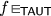, B proceeds as A and emulates the behavior of the XCA deciding $$\textsf {TAUT}$$ (see Proposition 7); however, unlike A, the computation branches of B which evaluate to false reject immediately while it is those that evaluate to true that continue evaluating the input formula. As a result, if $$f \in \textsf {TAUT}$$, all branches of B evaluate to true and continue evaluating the input in a synchronous manner; otherwise, there is a branch evaluating to false, and, since a single rejecting cell suffices for it to reject, B rejects immediately. The evaluation of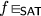is carried out analogously.

The modifications to A to obtain B do not impact its time complexity whatsoever; thus, B also has polynomial time complexity. $$\square$$

For the converse inclusion, consider the following $$\textsf {NP}$$ analogue of the $$\textsf {STATE}_\forall$$ language (cf. Definition 10 and Lemma 11):

### Definition 22

($$\textsf {STATE}_\exists$$) Let A be an XCA and V be the set of triples (wtz) as in Definition 10. $$\textsf {STATE}_\exists \subseteq V$$ is the subset of triples such that, for the input w, after t steps at least one of the active cells of A is in state z.

### Lemma 23

For any XCA A with polynomial time complexity, $$\textsf {STATE}_\exists (A) \in \textsf {NP}.$$

### Proof

Consider the NTM T from Lemma 11 and notice that, if any of the active cells of A in step $$\tau$$ have state z, then T will have at least one accepting branch; otherwise, none of the active cells of A in step $$\tau$$ have state z; thus, all branches of T are rejecting. $$\square$$

Using Lemma 23 to proceed as in Proposition 12 yields the following, from which Theorem 20 follows:

### Proposition 24

$$\textsf {EXCAP}\subseteq {\le _{tt}^p}(\textsf {NP})$$.

### One-cell-decision XCA

We turn to the discussion of XCAs whose acceptance condition is defined in terms of a distinguished cell which directs the automaton’s decision, considered the standard acceptance condition for CAs (Kutrib 2009). This condition is similar to the existential variant in the sense that the automaton’s termination is triggered by a single cell entering a final state. The difference is that, here, the position of this cell is fixed.

We consider only the case in which the decision cell is the leftmost active cell in the initial configuration (i.e., cell 0). By a one-cell-decision XCA (1XCA) we refer to an XCA which accepts if and only if 0 is in the accept state and rejects if and only if cell zero is in the reject state. Let $$\textsf {1XCAP}$$ denote the polynomial-time class of 1XCAs.

The position of the decision cell is fixed; with a polynomial-time restriction in place, it can only communicate with cells which are a polynomial (in the length of the input) number of steps apart. As a result, despite a 1XCA being able to efficiently increase its number of active cells exponentially (see Lemma 5), any cells impacting its decision must be at most a polynomial number of cells away from the decision cell. Thus:

### Theorem 25

$$\textsf {1XCAP}= \textsf {P}$$.

### Proof

The inclusion $$\textsf {1XCAP}\supseteq \textsf {P}$$ is trivial. For the converse, recall the construction of the NTM T in Lemma 11. T can be modified so that it works deterministically and always chooses the next configuration $$c_{i+1}$$ from $$c_i$$ by selecting cell zero as the middle cell. If cell zero is accepting, then T accepts immediately; if it is rejecting, then T also rejects immediately. This yields a simulation of a 1XCA by a (deterministic) TM which is only polynomially slower, thus implying $$\textsf {1XCAP}\subseteq \textsf {P}$$. $$\square$$

## Conclusion

This paper summarized the results of Modanese (2018) and also presented related and previously unpublished results from Modanese (2016). The main result was the characterization $$\textsf {XCAP}= {\le _{tt}^p}(\textsf {NP})$$ (Theorem 8) in Sect. 3, which also gave an alternative characterization based on NTMs (Theorem 14). In Sect. 4, XCAs with multiple accept and reject states were shown to be equivalent to the original model (Theorem 18). Also in Sect. 4, two other variants based on varying acceptance conditions were considered: the existential (EXCA), in which a single, though arbitrary cell may direct the automaton’s response; and the one-cell-decision XCA (1XCA), in which a fixed cell does so. In the first case, it was shown that the polynomial-time class $$\textsf {EXCAP}$$ equals $$\textsf {XCAP}$$ (Theorem 20); in the latter, it was shown that the polynomial-time class $$\textsf {1XCAP}$$ of 1XCAs equals $$\textsf {P}$$ (Theorem 25).

This paper has covered some XCA variants with diverse acceptance conditions. A topic for future work might be considering further variations in this sense (e.g., XCAs whose acceptance condition is based on majority instead of unanimity, which appears to lead to a model whose polynomial-time class equals $$\textsf {PP}$$). Another avenue of research lies in restricting the capabilities of XCAs and analyzing the effects thereof (e.g., restricting 1XCAs or SXCAs to a polynomial number of cells). A final open question is determining what polynomial speedups, if any, 1XCAs provide with respect to 1CAs.

## Change history

1. 1.

Strictly speaking, the codomain of $$\varPhi$$ (as here defined) is not only $$Q^{\mathbb {Z}}$$ but actually larger (since, for $$c \in Q^{\mathbb {Z}}$$ arbitrary, $$\varPhi (c)(z)$$ may be undefined for certain $$z \in {\mathbb {Z}}$$). However, since the configurations that arise in our context of XCAs have infinitely many occurrences of q in either direction (i.e., $$c(i) = q$$ holds for infinitely many $$i > 0$$ as well as infinitely many $$i < 0$$), in this case $$\varPhi (c)(z)$$ is guaranteed to be defined for every $$z \in {\mathbb {Z}}$$, that is, $$\varPhi (c) \in Q^{\mathbb {Z}}$$. Hence, to simplify the presentation, we write only “$$Q^{\mathbb {Z}}$$” here.

2. 2.

For example, have each cell contain a bit counter and, if needed, wait for one step before transitioning to an accept or reject state.

3. 3.

The main reason for this is that our construction of T non-deterministically picks cells starting at the initial configuration of A instead of (picking a final computation step and then) an arbitrary cell from the final configuration. The issue with the latter approach is that then, in order to compute the chosen cell’s state, we would require a procedure that, given an arbitrary cell z in the final configuration of A and without simulating A directly, determines whether z was already present in the initial configuration and, provided it was not, in which step exactly did it turn from a hidden cell into an active one. This is indeed feasible if we constructed A ourselves but virtually impossible in case A is arbitrary (which is the setting of the proof).

## References

1. Arora S, Barak B (2009) Computational complexity: a modern approach. Cambridge University Press, Cambridge

2. Arrighi P, Dowek G (2013) Causal graph dynamics. Inf Comput 223:78–93

3. Buss SR, Hay L (1991) On truth-table reducibility to SAT. Inf Comput 91(1):86–102

4. Cook SA (1971) The complexity of theorem- proving procedures. In: Proceedings of the 3rd annual ACM symposium on theory of computing, May 3–5, 1971, Shaker Heights, Ohio, USA, pp 151–158

5. Dantchev SS (2008) Dynamic neighbourhood cellular automata. In: Visions of computer science-BCS international academic conference, Imperial College, London, UK, 22–24 September 2008, pp 60–68

6. Delorme M, Mazoyer J (eds) (1999) Cellular automata: a parellel model. Mathematics and its applications 460. Springer, Amsterdam

7. Dubacq J-C (1994) Different kinds of neighborhood-varying cellular automata. Maîtrise (honors bachelor’s) thesis. École normale superiéure de Lyon

8. Gordillo JL, Luna JV (1994) Parallel sort on a linear array of cellular automata. In Proceedings of the IEEE international conference on systems, man and cybernetics, SMC 1994, San Antonio, Texas, USA, October 2–5, 1994, pp 1903–1907

9. Ibarra OH, Palis MA, Kim SM (1985) Fast parallel language recognition by cellular automata. Theor Comput Sci 41:231–246

10. Ilachinski A, Halpern P (1987) Structurally dynamic cellular automata. Complex Syst 1(3):503–527

11. Kim S, McCloskey R (1990) A characterization of constant-time cellular automata computation. Phys D 45(1–3):404–419

12. Kutrib M (2009) Cellular automata and language theory. In: Encyclopedia of complexity and systems science, pp 800–823

13. Kutrib M, Malcher A, Wendlandt M (2015) Shrinking one-way cellular automata. In: Cellular automata and discrete complex systems-21st IFIP WG 1.5 international workshop, AUTOMATA 2015, Turku, Finland, June 8–10, 2015. Proceedings, pp 141–154

14. Ladner RE, Lynch NA (1976) Relativization of questions about log space computability. Math Syst Theory 10:19–32

15. Ladner RE, Lynch NA, Selman AL (1975) A comparison of polynomial time reducibilities. Theor Comput Sci 1(2):103–123

16. Modanese A (2016) Shrinking and expanding one-dimensional cellular automata. Bachelor’s thesis. Karlsruhe Institute of Technology (KIT)

17. Modanese A (2018) Complexity-theoretical aspects of expanding cellular automata. Master’s thesis. Karlsruhe Institute of Technology (KIT)

18. Modanese A (2019) Complexity-theoretic aspects of expanding cellular automata. In: Cellular automata and discrete complex systems-25th IFIP WG 1.5 international workshop, AUTOMATA 2019, Guadalajara, Mexico, June 26–28, 2019, Proceedings, pp 20–34

19. Modanese A, Worsch T (2016) Shrinking and expanding cellular automata. In: Cellular automata and discrete complex systems-22nd IFIP WG 1.5 international workshop, AUTOMATA 2016, Zurich, Switzerland, June 15–17, 2016, Proceedings, pp 159–169

20. Rosenfeld A (1979) Picture languages: formal models for picture recognition. Academic Press, London

21. Rosenfeld A, Wu AY (1981) Reconfigurable cellular computers. Inf Control 50(1):60–84

22. Rosenfeld A, Wu AY, Dubitzki T (1983) Fast language acceptance by shrinking cellular automata. Inf Sci 30(1):47–53

23. Smith AR III (1971) Simple computation-universal cellular spaces. J ACM 18(2):339–353

24. Sommerhalder R, van Westrhenen SC (1983) Parallel language recognition in constant time by cellular automata. Acta Inf 19:397–407

25. Tomita K, Kurokawa H, Murata S (2002) Graph automata: natural expression of self-reproduction. Phys D 171(4):197–210

26. Wagner KW (1990) Bounded query classes. SIAM J Comput 19(5):833–846

## Acknowledgements

I thank Thomas Worsch for his mentoring, encouragement, and support during the writing of this paper. I would also like to thank Dennis Hofheinz for pointing out a crucial mistake in a preliminary version of this paper as well as the anonymous referees for their valuable remarks and suggestions. Open Access funding enabled and organized by Projekt DEAL.

## Funding

Open Access funding enabled and organized by Projekt DEAL.

## Author information

Authors

### Corresponding author

Correspondence to Augusto Modanese.

### Publisher's Note

Springer Nature remains neutral with regard to jurisdictional claims in published maps and institutional affiliations.

The original online version of this article was revised: In the original publication, certain mathematical formulas were rendered incorrectly or are missing from the text.

Parts of this paper have been submitted (Modanese 2016, 2018) in partial fulfillment of the requirements for the degrees of Bachelor of Science and Master of Science at the Karlsruhe Institute of Technology (KIT).

## Rights and permissions

Open Access This article is licensed under a Creative Commons Attribution 4.0 International License, which permits use, sharing, adaptation, distribution and reproduction in any medium or format, as long as you give appropriate credit to the original author(s) and the source, provide a link to the Creative Commons licence, and indicate if changes were made. The images or other third party material in this article are included in the article's Creative Commons licence, unless indicated otherwise in a credit line to the material. If material is not included in the article's Creative Commons licence and your intended use is not permitted by statutory regulation or exceeds the permitted use, you will need to obtain permission directly from the copyright holder. To view a copy of this licence, visit http://creativecommons.org/licenses/by/4.0/.

Reprints and Permissions